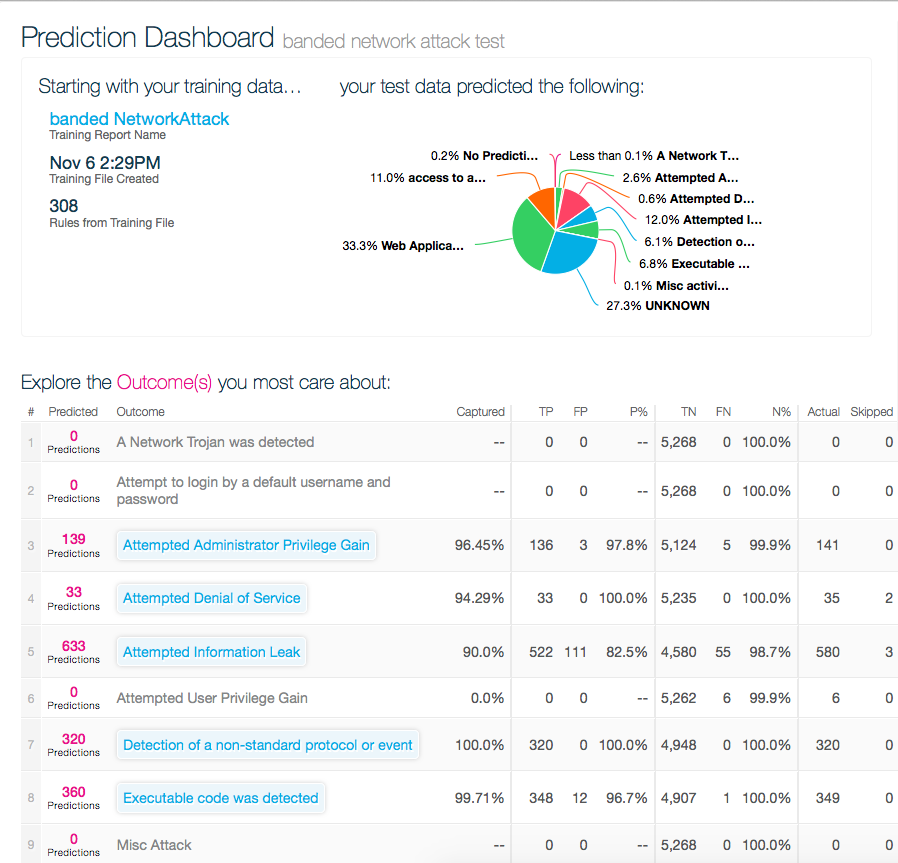Prediction DashboardPrediction Dashboard

The predictions page is helpful to see how accurate the engine is at making predictions, and displays statistics on the engine's performance. The columns are defined below:Captured- This is the percentage of predictions that the engine made for the item that the engine was able to correctly predict.

TP- True Positives, this is the number of times the engine predicted an item would occur, and it did occur (e.g. engine predicted “sick” and the patient was sick).

FP- False Positives, this is the number of times the engine predicted an item would occur and the item did not occur (e.g. engine predicted “sick” and the patient was not sick).

P%– The number of True Positives divided by the total number of time the engine predicted a positive outcome, displayed as a percentage.

TN- True Negatives, the number of times the engine confidently predicted the item would not occur in a transaction and was right (e.g. engine predicted healthy people were not sick).

FN- False Negatives, the number of times the engine confidently predicted the item would not occur in a transaction and the item actually was in the transaction. (e.g. engine predicted healthy people were sick).

N%- similar to P%, this is the number of True Negatives divided by Total Negatives.

Actual- The actual number of times the item occurred in the data set.

Skip- The number of times the engine did not have enough information to confidently list an outcome.